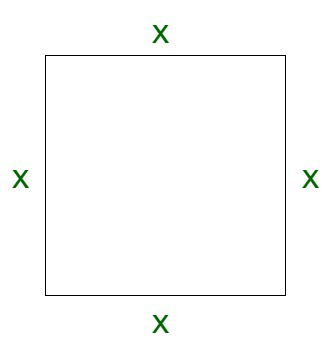# What happens to the area of a square when its side is tripled?

• Last Updated : 02 Nov, 2021

A square is a regular quadrilateral. It has all four edges of equal length. In addition to this, all four angles are also equal in nature. The angles of the square subtend an angle equivalent to 90 degrees. The diagonals of the square are equal and bisect each other at an angle of 90 degrees. A square is a special case of the rectangle where the opposite pair of sides are equal in length.

Properties of a Square

A square has the following important properties :

• A square has 4 vertices and 4 edges
• Opposite sides of the square are parallel to each other
• Diagonals are equal to each other.
• Diagonals of the square bisect each other at 90°
• The length of diagonals is more than the corresponding sides of the square
• All of the interior angles are equivalent to 90° each.

### What happens to the area of a square when its side is tripled?

Solution:

Since, we know,

Area of a square = (side)2

Let us assume the side of the square to be x and the area to be denoted by A.

On substituting the values, we get,

A = x2

If we triple the side, the side of the square each becomes 3x.

Now, the new area, denoted by A’ is given by,

A’ = (3x)2

A’ = 9x2

On comparing both the areas, we get,

A’/A = 9x2 / x2

= 9

Therefore, on tripling the side, the area of the square becomes 9 times the original.### Sample Questions

Question 1: If a side is made n times, how many times the area is increased?

Solution:

Since, we know,

Area of a square = (side)2

Modified area of a square,

New area of square = (n*side)2

On comparing, we obtain, the area increases by n2 times.

Question 2: By the upper formula, what happens to the area of a square if the side is reduced to half.

Solution:

By straight calculation of the area of a square through its side, the area of the square is reduced and it becomes one-fourth.

This is because,

Original Area = side2

New Area = (1/2 side)2

New Area = 1/4 * Original Area

Question 3: If the area becomes 8 times, how many times is the side of the square affected?

Solution:

Let us assume the side of the square to be s.

Since, we know,

Area of a square A = s2

Now, the New area, A’ of the square is 8 times.

Therefore,

A’ = 8A

Let us assume the side of the new square to be s’.

s’2 = 8s2

Taking square root on both sides, we have,

s’ = 2√2 s

Therefore, side of the square becomes 2√2 times.

Question 4: What happens to the area of a square when its side is doubled?

Solution:

Area of a square = (side)2

Let us assume the side of the square to be x and the area to be denoted by A.

On substituting the values, we get,

A = x2

If we double the side, the side of the square each becomes 2x.

Now, the new area, denoted by A’ is given by,

A’ = (2x)2

A’ = 4x2

On comparing both the areas, we get,

A’/A = 4x2 / x2

= 4

My Personal Notes arrow_drop_up